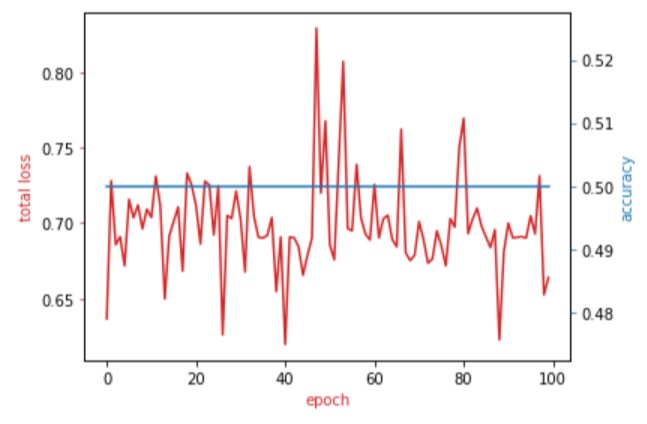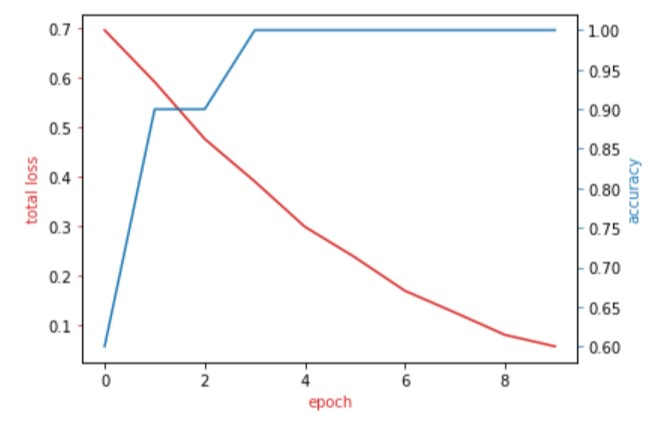# Given groups=1, weight of size [6, 1, 3, 3], expected input[13, 3, 100, 100] to have 1 channels, but got 3 channels instead

I am new to pytorch and I am trying to build a cnn model using the following code. My issue is I dont know how to calculate input and output parameters. my images are of 100x 100 and grayscale. more my dataset is of 1690 images and I want to batch them in 13 images. there two output sets pos or neg. Can someone please explain me how to give values of command between two # lines

class Net(nn.Module):

``````def __init__(self):
super(Net, self).__init__()
############################
self.conv1 = nn.Conv2d(1, 6, 3)
self.conv2 = nn.Conv2d(6, 16, 3)
# an affine operation: y = Wx + b
self.fc1 = nn.Linear(16 * 5 * 5, 120)
self.fc2 = nn.Linear(120, 84)
self.fc3 = nn.Linear(84, 2)
``````

########################################
def forward(self, x):
# Max pooling over a (2, 2) window
x = F.max_pool2d(F.relu(self.conv1(x)), (2, 2))
# If the size is a square you can only specify a single number
x = F.max_pool2d(F.relu(self.conv2(x)), 2)
x = x.view(-1, self.num_flat_features(x))
x = F.relu(self.fc1(x))
x = F.relu(self.fc2(x))
x = self.fc3(x)
return x

``````def num_flat_features(self, x):
size = x.size()[1:]  # all dimensions except the batch dimension
num_features = 1
for s in size:
num_features *= s
return num_features
``````

net = Net()
print(net)

n_epochs=3
loss_list=[]
accuracy_list=[]
N_test=len(validation_dataset)

def train_model(n_epochs):
for epoch in range(n_epochs):
z = net(x)
loss = criterion(z, y)
loss.backward()
optimizer.step()

``````    correct=0
#perform a prediction on the validation  data
z = net(x_test)
_, yhat = torch.max(z.data, 1)
correct += (yhat == y_test).sum().item()
accuracy = correct / N_test
accuracy_list.append(accuracy)
loss_list.append(loss.data)
``````

train_model(n_epochs)

Conv layers have a few mandatory arguments, i.e. `in_channels`, `out_channels`, and `kernel_size`.
Other arguments like `stride` and `padding` are optional.
A short explanation:

• `in_channels`: the number of input channels of the activation volume to the current conv layer. In case it’s the first layer, you have to set it to the number of channels of your input image. In your case, as you are dealing with grayscale images, you would have to set it to `1`.
• `out_channels`: number of filter kernels in your conv layer. Each filter kernel creates a channel in the output activation volume. The first conv layer has 6 kernels and outputs an activation of `[batch_size, 6, h, w]`. This also means the following conv layer should use `in_channels=6`.
• `kernel_size`: the spatial size of your kernel (height and width). If only a single value is specified, both the height and width will be set to this value.

If you want to pass your conv output to a linear layer, you should flatten it in the standard use case.
Your `fc1` layer takes `in_features=16*5*5`, which means the activation being passed to it is of shape `[batch_size, 16, 5, 5]`. Similar to the conv layers, the `out_features` of one linear layers define the `in_features` of the subsequent one, if no other (pooling) operation is performed.

For an input size of `[1, 100, 100]`, you’ll get an error, since the `in_features` of `self.fc1` won’t match the activation shape, and you should specify it as `in_features=16 * 23 * 23`.

Have a look at Stanfrod’s CS231n - CNN section where all shapes and operations are beautifully explained and let me know if you need some more information.

PS: You can add code snippets using three backticks `2 Likes

Thank you so much . This has been very cognizant…

Thank You for your previous solution. But there are 2 issues.
First of all Images in my dataset are in grayscale but when I feed them I have to keep input channel as 3. Otherwise it wont work.

secondly I have been running the model multiple times but my accuracy has not increased I have tried 3, 10 and 100 epochs.I guess all three channels have the same values then. You could check if that’s the case and just keep a single channel, since the other two won’t give you any more information, if you would like to train from scratch.

Regarding your loss curves, I would try to overfit a small subset of your dataset (e.g. 1-10 samples) and see if that’s working. If your model can’t fit this small sample, you might have some other bugs in your code. However, if it’s working, you could try to carefully scale up your experiment and play with some hyperparameters.

1 Like

Can you explain the second point in a simple sense…

Sure!
Based on your loss curves it looks like some hyperparameters might be a bit off (e.g. the learning rate might be too high). One approach would be to use your whole data and the model as it is now and to play around with the hyperparameters.

However, it might also be useful to check for some code bugs (e.g. forgetting to zero out the gradients etc.).
An easy way to check for code sanity is to use a very small subset of your data, e.g. just 10 samples (10 image - target pairs), and try to train your model on this small dataset. If your model can successfully overfit the data, it’s a good sign, as you’ll see that the code seems to work and the model architecture might be alright to learn the data distribution.
On the other hand, if your model can’t learn even these 10 samples, you should check for code bugs (or post your code in this forum so that we can help you debugging).Let me know, if this makes it clearer.

1 Like

As you said I used only 10 samples. On changing my learning rate from 0.1 to 0.001 I was able to get a better loss curve..

Looks good!
Now you could try to scale up the experiment a bit and play around with some more hyperparameters to get such a nice loss curve with the whole dataset.

This has been very helpful. I am utterly thankful. The link which you provided for understanding different shapes was also very informative.
Graph with 10 epoch and 0.001lrI am getting these error. If I try saving it in any other drive then my error would be permission denied. In that case also I have changed permission but it was of no use.

I have understood my error, I have to name the model also with it. But I am still having problems in loading the model. I have been searching and trying various methods but none have been useful.

What I am doing wrong over here??? !And in tutorial for Saving and Loading model they have mentioned not to give paths rather deserialize the parameters. What does that mean ?

You have to create an instance of your model first, then load the `state_dict`:

``````net = MyModel()
I just used `MyModel()` as a placeholder. You should use the class name you have defined.Based on your first post it looks like your class is named `Net()`.